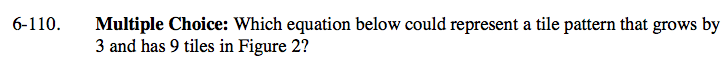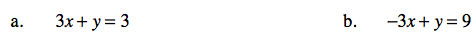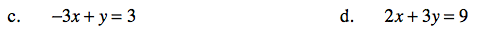### Home > CAAC > Chapter 6 > Lesson 6.3.1 > Problem6-110

6-110.
1. Multiple Choice: Which equation below could represent a tile pattern that grows by 3 and has 9 tiles in Figure 2? Homework Help ✎

1. 3x + y = 3

2. −3x + y = 9

3. −3x + y = 3

4. 2x + 3y = 9Work backwards from Figure 2 to find the starting value of tiles at Figure 0, then write the rule and match your answer.

(c) −3x + y = 3